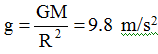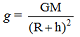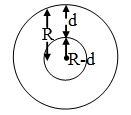# Physics

### Chapter : 4. Gravitation

#### Variation in the value of Gravitational Acceleration (g)

(A) Variation with altitude or height :
When a body moves above the earth's surface the distance of the body from the centre of earth increases there by decreasing the force of attraction.; at the earth's surface.; at a height h above the earth's surface.
As we go above the earth's surface the value of g goes on decreasing.(B) Variation with depth d :
As we go deeper inside the earth, the body gets attracted by the core of the earth which is smaller in mass.As we go inside the earth, the value of g decreases.
Force of attraction decreases and thus decreasing the value of g and becoming zero at the centre.

(C) Variation due to rotation of the earth :
Due to the rotation of the earth, the weight of a body is maximum at the poles and minimum at the equator.